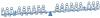BrainDen.com - Brain Teasers

## Question1) How can the you balance the see-saw by exchanging any 2 kids position 4 times?
2) Which twin weighs different from others?

## Recommended Posts

• 0

Swap the red kids:

T T L + E O E V N N ^ W W E O V E + L E E
E T L + E O E V N N ^ W W E O V E + L T E
E T L + E O E V W N ^ N W E O V E + L T E
E T L + E V E O W N ^ N W E O V E + L T E
E T L + E V E O W N ^ N W O E V E + L T E <= final balanced position

Which twins weight different?

If the last three swaps are done first, we have this position

T T L + E V E O W N ^ N W O E V E + L E E,

which is symmetrical except for the outer T T vs E E.

If this still unbalanced to the left, and I don't see

how we could conclude that, then we could conclude

that TT are heavier than EE.

But since OP asks only to identify difference from all

others, not one heavier or lighter than another, then

a different configuration likely holds the answer.

##### Share on other sites

• 0

from left side to right side; left to right:

switch the second T with the first W

switch the first L with the first O

switch the first O with the first L

switch the first N with the third E

##### Share on other sites

• 0

That is it ! Bonanova's arrangement got it balanced. That is the same symmetric solution I got.
But unlike odd weight balance scale problem, this one has a predetermined odd weight and
should have a 'leading clue' i.e. not symmetric - the anagramatist equation . Plainglazed solution shows:
T W O + E L E V E N = T W E L V E + O N E all E's are same weights , so only the O's are odds then.
Thanks for solving..more power !

## Join the conversation

You can post now and register later. If you have an account, sign in now to post with your account.×   Pasted as rich text.   Paste as plain text instead

Only 75 emoji are allowed.

×   Your previous content has been restored.   Clear editor

×   You cannot paste images directly. Upload or insert images from URL.